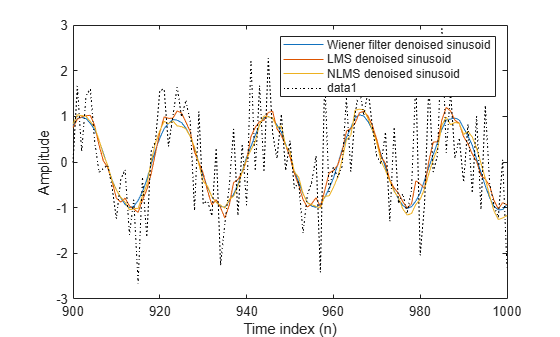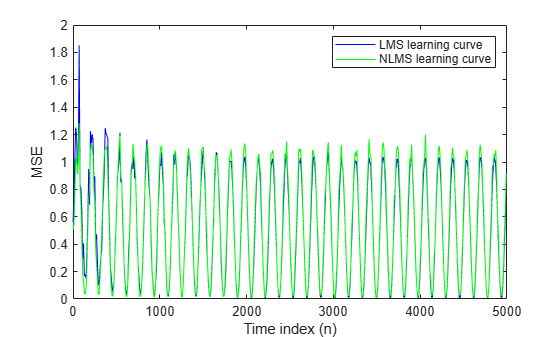Documentation

## Signal Enhancement Using LMS Algorithm and Normalized LMS Algorithm

This example illustrates one way to use a few of the adaptive filter algorithms provided in the toolbox. In this example, a signal enhancement application is used as an illustration. While there are about 30 different adaptive filtering algorithms included with the toolbox, this example demonstrates two algorithms — least means square (LMS) and normalized LMS. Both of these algorithms are available with the dsp.LMSFilter System object fallback for tm_systemobject .

### Create the Signals for Adaptation

The goal is to use an adaptive filter to extract a desired signal from a noise-corrupted signal by filtering out the noise. The desired signal (the output from the process) is a sinusoid with 1000 samples.

```n = (1:1000)'; s = sin(0.075*pi*n);```

To perform adaptation requires two signals:

• a reference signal

• a noisy signal that contains both the desired signal and an added noise component.

#### Generate the Noise Signal

To create a noise signal, assume that the noise v1 is autoregressive, meaning that the value of the noise at time t depends only on its previous values and on a random disturbance.

```v = 0.8*randn(1000,1); % Random noise part. ar = [1,1/2]; % Autoregression coefficients. v1 = filter(1,ar,v); % Noise signal. Applies a 1-D digital % filter.```

#### Corrupt the Desired Signal to Create a Noisy Signal

To generate the noisy signal that contains both the desired signal and the noise, add the noise signal v1 to the desired signal s. The noise-corrupted sinusoid x is

`x = s + v1;`

where s is the desired signal and the noise is v1. Adaptive filter processing seeks to recover s from x by removing v1. To complete the signals needed to perform adaptive filtering, the adaptation process requires a reference signal.

#### Create a Reference Signal

Define a moving average signal v2 that is correlated with v1. This v2 is the reference signal for the examples.

```ma = [1, -0.8, 0.4 , -0.2]; v2 = filter(ma,1,v);```

Two similar adaptive filters — LMS and NLMS — form the basis of this example, both sixth order. Set the order as a variable in MATLAB fallback for tm_matlab and create the filters.

```L = 7; lms = dsp.LMSFilter(L,'Method','LMS'); nlms = dsp.LMSFilter(L,'Method','Normalized LMS');```

### Choose the Step Size

LMS-like algorithms have a step size that determines the amount of correction applied as the filter adapts from one iteration to the next. Choosing the appropriate step size is not always easy, usually requiring experience in adaptive filter design.

A step size that is too small increases the time for the filter to converge on a set of coefficients. This becomes an issue of speed and accuracy.One that is too large may cause the adapting filter to diverge, never reaching convergence. In this case, the issue is stability — the resulting filter might not be stable.

As a rule of thumb, smaller step sizes improve the accuracy of the convergence of the filter to match the characteristics of the unknown, at the expense of the time it takes to adapt.

The maxstep function of dsp.LMSFilter object determines the maximum step size suitable for each LMS adaptive filter algorithm that still ensures that the filter converges to a solution. Often, the notation for the step size is µ.

`[mumaxlms,mumaxmselms] = maxstep(lms,x)`
```mumaxlms = 0.2088 ```
```mumaxmselms = 0.1214 ```
`[mumaxnlms,mumaxmsenlms] = maxstep(nlms,x)`
```mumaxnlms = 2 ```
```mumaxmsenlms = 2 ```

### Set the Adapting Filter Step Size

The first output of maxstep is the value needed for the mean of the coefficients to converge while the second is the value needed for the mean squared coefficients to converge. Choosing a large step size often causes large variations from the convergence values, so choose smaller step sizes generally.

`lms.StepSize = mumaxmselms/30 `
```lms = dsp.LMSFilter with properties: Method: 'LMS' Length: 7 StepSizeSource: 'Property' StepSize: 0.0040 LeakageFactor: 1 InitialConditions: 0 AdaptInputPort: false WeightsResetInputPort: false WeightsOutput: 'Last' Show all properties ```
`nlms.StepSize = mumaxmsenlms/20 `
```nlms = dsp.LMSFilter with properties: Method: 'Normalized LMS' Length: 7 StepSizeSource: 'Property' StepSize: 0.1000 LeakageFactor: 1 InitialConditions: 0 AdaptInputPort: false WeightsResetInputPort: false WeightsOutput: 'Last' Show all properties ```

### Filter with the Adaptive Filters

Now you have set up the parameters of the adaptive filters and you are ready to filter the noisy signal. The reference signal, v2, is the input to the adaptive filters. x is the desired signal in this configuration.

Through adaptation, y, the output of the filters, tries to emulate x as closely as possible.

Since v2 is correlated only with the noise component v1 of x, it can only really emulate v1. The error signal (the desired x), minus the actual output y, constitutes an estimate of the part of x that is not correlated with v2 — s, the signal to extract from x.

```[ylms,elms,wlms] = lms(v2,x); [ynlms,enlms,wnlms] = nlms(v2,x);```

### Compute the Optimal Solution

For comparison, compute the optimal FIR Wiener filter.

```bw = firwiener(L-1,v2,x); % Optimal FIR Wiener filter yw = filter(bw,1,v2); % Estimate of x using Wiener filter ew = x - yw; % Estimate of actual sinusoid```

### Plot the Results

Plot the resulting denoised sinusoid for each filter — the Wiener filter, the LMS adaptive filter, and the NLMS adaptive filter — to compare the performance of the various techniques.

```plot(n(900:end),[ew(900:end), elms(900:end),enlms(900:end)]); legend('Wiener filter denoised sinusoid',... 'LMS denoised sinusoid','NLMS denoised sinusoid'); xlabel('Time index (n)'); ylabel('Amplitude');```

As a reference point, include the noisy signal as a dotted line in the plot.

```hold on plot(n(900:end),x(900:end),'k:') xlabel('Time index (n)'); ylabel('Amplitude'); hold off```### Compare the Final Coefficients

Finally, compare the Wiener filter coefficients with the coefficients of the adaptive filters. While adapting, the adaptive filters try to converge to the Wiener coefficients.

`[bw.' wlms wnlms]`
```ans = 7×3 1.0273 0.8761 1.0202 0.3390 0.1368 0.3225 0.1201 0.0095 0.1227 0.0425 -0.0038 0.0495 0.1098 0.0503 0.0773 0.0534 -0.0094 0.0295 0.0146 -0.0054 -0.0179 ```

### Reset the Filter Before Filtering

You can reset the internal filter states at any time by calling the reset method on the filter object.

For instance, the following successive calls produce the same output after resetting the object.

```[ylms,elms,wlms] = lms(v2,x); [ynlms,enlms,wnlms] = nlms(v2,x);```

If you do not reset the filter object, the filter uses the final states and coefficients from the previous run as the initial conditions for the next run and set of data.

### Investigate Convergence Through Learning Curves

To analyze the convergence of the adaptive filters, look at the learning curves. The toolbox provides methods to generate the learning curves, but you need more than one iteration of the experiment to obtain significant results.

This demonstration uses 25 sample realizations of the noisy sinusoids.

```n = (1:5000)'; s = sin(0.075*pi*n); nr = 25; v = 0.8*randn(5000,nr); v1 = filter(1,ar,v); x = repmat(s,1,nr) + v1; v2 = filter(ma,1,v);```

### Compute the Learning Curves

Now compute the mean-square error. To speed things up, compute the error every 10 samples.

First, reset the adaptive filters to avoid using the coefficients it has already computed and the states it has stored.

```reset(lms); reset(nlms); M = 10; % Decimation factor mselms = msesim(lms,v2,x,M); msenlms = msesim(nlms,v2,x,M); %plot(1:M:n(end),[mselms,msenlms]) plot(1:M:n(end),mselms,'b',1:M:n(end),msenlms,'g') legend('LMS learning curve','NLMS learning curve') xlabel('Time index (n)'); ylabel('MSE');```In the next plot you see the calculated learning curves for the LMS and NLMS adaptive filters.

### Compute the Theoretical Learning Curves

For the LMS and NLMS algorithms, functions in the toolbox help you compute the theoretical learning curves, along with the minimum mean-square error (MMSE) the excess mean-square error (EMSE) and the mean value of the coefficients.

MATLAB fallback for tm_matlab may take some time to calculate the curves. The figure shown after the code plots the predicted and actual LMS curves.

```reset(lms); [mmselms,emselms,meanwlms,pmselms] = msepred(lms,v2,x,M); x = 1:M:n(end); y1 = mmselms*ones(500,1); y2 = emselms*ones(500,1); y3 = pmselms; y4 = mselms; plot(x,y1,'m',x,y2,'b',x,y3,'k',x,y4,'g') legend('MMSE','EMSE','predicted LMS learning curve',... 'LMS learning curve') xlabel('Time index (n)'); ylabel('MSE');```Watch now